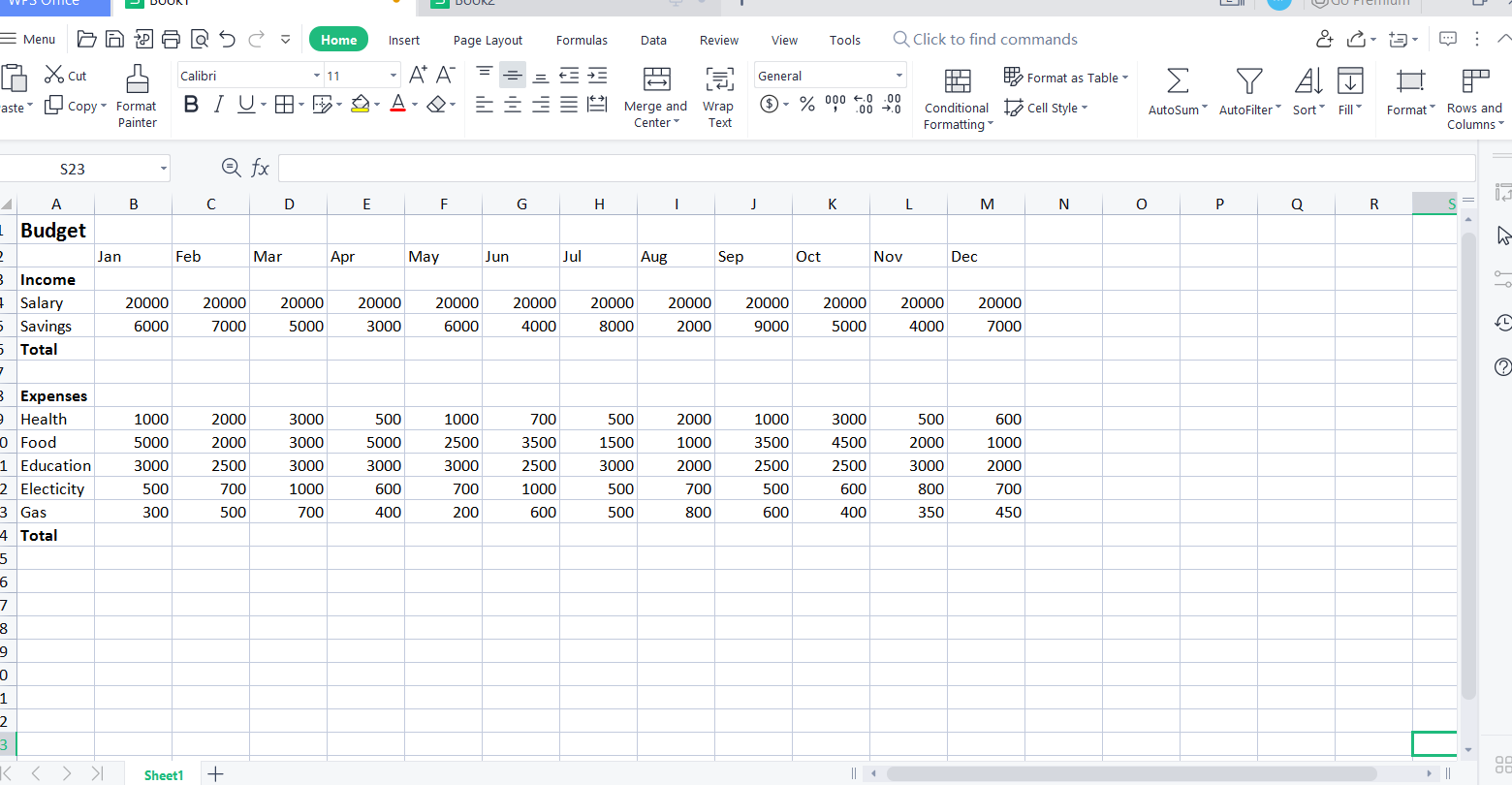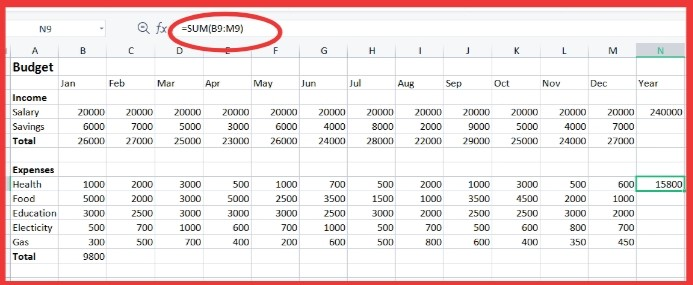# How to calculate monthly budget in excel?

July 22, 2022
2.8K Views
0

A free Office suite fully compatible with Microsoft Office

A free Office suite fully compatible with Microsoft Office

Most of the people are in the grip of financial crisis due to poor budget planning. Overlapping debts, taxes, paid and unpaid bills create unpleasant circumstances which become hard to handle. To avoid such distressing situation one should manage their expenses properly by creating a suitable budget plan.

Every business person as well as a lay man has to go through an uncomfortable time in making a proper budget plan. This process is very exhausting for both mind and body. Fortunately, Excel has introduced a feature for the calculation of budget which has provided an easy hand to the people. Excel consists of a spreadsheet in which all sort of financial data can be stored in an organized manner. In this article we will discuss some important and easy steps to calculate budget in excel. These tips are applicable for 2016\2019 versions.

How to calculate monthly budget in excel

To calculate monthly budget in excel first we have to organize our budget properly on spreadsheet. During organizing budget we will apply some formulas which will make the managing was budget quite simple.

This process works with 2016\2019 versions.

1. First, we will create a proper monthly budget plan on our excel spreadsheet.2. After entering our budget data we will calculate the total income of every month.

3. We will enter the formula =SUM(B2:B5) then we will apply the formula to range C6 to M6.

4. In this way we will calculate total income of each and every month.

5. We will apply the same formula to calculate the total amount of expenses.

6. We will calculate the total amount of each budget by using formula= Sum(B9:M9) and apply the formula to the range N9 to N13.How to calculate yearly budget in excel

To calculate yearly budget we will add the sum of the entire amount of all the months. The formula that is applied is same as the above mentioned formula.

This method works with 2016\2016 versions.

1. For creating yearly budget, we will use the formula IF(SUM(N4:N5)=SUM(B6:M6),SUM(N4:N5),FALSE),

1. Then we will calculate the surplus of yearly budget by entering the formula= B6-B14 and we will apply this formula in range C17 to N17.

In the above method we have briefly discussed the calculation of yearly budget

How to calculate budget using charts.

There are different charts in excel that can make the representation of data more clear and easy to comprehend we can add certain charts in our spreadsheet by simply clicking the option of insert then we will click the option of charts. We can also represent our budget in form of graphs. Graphical representation is a more classic way to organize data.

This method works with 2016\2019 versions.

1. First, we will select the cells A4 to A5 then we will insert a pie chart.

1. For cells A9 to A13 we will insert graphs.

1. For correct representation we will label the graph and pie chart.

Lastly we will save our budget file in .xltx format.